## Constraint fromTheoscillation frequency, which is related to the mass difference between the light and the heavy mass eigenstates of the system, is proportional toin the Standard Model. Neglecting terms giving small contributions,is independent ofand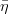. Instead, the ratio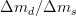is proportional toandand the advantage of using this ratio instead of onlyis that the ratio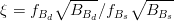is expected to be better determined from the theory than the individual quantities entering its expression. The measurement ofgives a similar constraint as:In practice a problem arises in the (possible) use of the information fromtwice in the fit: in theconstraint itself and in the ratio. The correlation has to be taken into account. One should recall thatalone would give a constraint on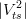and so. It should also be considered that due to the error coming from chiral extrapolations the quantities which are best known and which are effectively calculated areandwhileis derived from the other two. For this reason we write the constraints in the following way :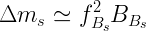Here the quantities entering in the expression ofare the calculated ones:and. To make the constraint onmore effective both quantities have to be improved. The constraint onhelps in improving the knowledge of one of those:. We implemented this bound using directly the information from the experimental likelihood provided by CDF. The representation of this constraint in theplane is given below.EPS - PDF - PNG - JPG - GIF

Ideas, requests, problems regarding this web site? Send feedback